{{announcement.body}}
{{announcement.title}}

# Classification From Scratch, Part 5 of 8: Penalized Lasso Logistic

DZone 's Guide to

# Classification From Scratch, Part 5 of 8: Penalized Lasso Logistic

### In this post, we discuss penalization based on the so-called Lasso regression, and how to code these algorithms in R. Read on to get started!

· Big Data Zone ·
Free Resource

Comment (0)

Save
{{ articles.views | formatCount}} Views

This is the fifth post of our series on classification from scratch, following the previous post on penalization using the ℓ2 norm (so-called Ridge regression), this time, we will discuss penalization based on the ℓ1 norm (the so-called Lasso regression).

First of all, one should admit that if the name stands for least absolute shrinkage and selection operator, that’s actually a very cool name… Funny story, a few years before Lasso was introduced, Leo Breiman introduced the Garrote Technique… “The garrote eliminates some variables, shrinks others, and is relatively stable.”I guess that somehow, the Lasso is the extension of the Garotte Technique.

## Normalization of the Covariates

As we previously discussed, the first step will be to consider linear transformations of all covariates xj to get centered and scaled variables (with unit variance):

y = myocarde$PRONO X = myocarde[,1:7] for(j in 1:7) X[,j] = (X[,j]-mean(X[,j]))/sd(X[,j]) X = as.matrix(X) ## Ridge Regression (from scratch) The heuristics about Lasso regression is the following graph. In the background, we can visualize the (two-dimensional) log-likelihood of the logistic regression, and the blue square is the constraints we have if we rewite the optimization problem as a constrained optimization problem: LogLik = function(bbeta){ b0=bbeta beta=bbeta[-1] sum(-y*log(1 + exp(-(b0+X%*%beta))) - (1-y)*log(1 + exp(b0+X%*%beta)))} u = seq(-4,4,length=251) v = outer(u,u,function(x,y) LogLik(c(1,x,y))) image(u,u,v,col=rev(heat.colors(25))) contour(u,u,v,add=TRUE) polygon(c(-1,0,1,0),c(0,1,0,-1),border="blue")The nice thing here is that is works as a variable selection tool, since some components can be null here. That’s the idea behind the following (popular) graph(with Lasso on the left, and Ridge on the right) Heuristically, the mathematical explanation is the following. Consider a simple regression yi=xiβ+ε, with ℓ1-penality and a ℓ2-loss function. The optimization problem becomes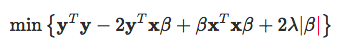The first order condition can be written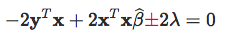Assuming that y^Tx>0, then the solution is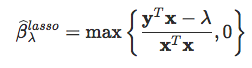(we get a corner solution when λ is large). ## Optimization Routine As in our previous post, let us start with standard (R) optimization routines, such as BFGS PennegLogLik = function(bbeta,lambda=0){ b0=bbeta beta=bbeta[-1] -sum(-y*log(1 + exp(-(b0+X%*%beta))) - (1-y)*log(1 + exp(b0+X%*%beta)))+lambda*sum(abs(beta)) } opt_lasso = function(lambda){ beta_init = lm(PRONO~.,data=myocarde)$coefficients
logistic_opt = optim(par = beta_init*0, function(x) PennegLogLik(x,lambda),
hessian=TRUE, method = "BFGS", control=list(abstol=1e-9))
logistic_opt$par[-1] } v_lambda=c(exp(seq(-4,2,length=61))) est_lasso=Vectorize(opt_lasso)(v_lambda) library("RColorBrewer") colrs=brewer.pal(7,"Set1") plot(v_lambda,est_lasso[1,],col=colrs,type="l") for(i in 2:7) lines(v_lambda,est_lasso[i,],col=colrs[i],lwd=2)But it is very heratic… or non stable. ## Using glmnet Just to compare, with R routines dedicated to Lasso, we get the following: library(glmnet) glm_lasso = glmnet(X, y, alpha=1) plot(glm_lasso,xvar="lambda",col=colrs,lwd=2)plot(glm_lasso,col=colrs,lwd=2)If we look carefully what’s in the ouput, we can see that there is variable selection, in the sense that some βj,λ=0, in the sense “really null.” glmnet(X, y, alpha=1,lambda=exp(-4))$beta
7x1 sparse Matrix of class "dgCMatrix"
s0
FRCAR  .
INCAR  0.11005070
INSYS  0.03231929
PRDIA  .
PAPUL  .
PVENT -0.03138089
REPUL -0.20962611

Of course, with out optimization routine, we cannot expect to have null values

opt_lasso(.2)
FRCAR         INCAR         INSYS         PRDIA
0.4810999782  0.0002813658  1.9117847987 -0.3873926427
PAPUL         PVENT        REPUL
-0.0863050787 -0.4144139379 -1.3849264055

So clearly, it will be necessary to spend more time today, to understand how it works.

## Orthogonal Covariates

Before getting into the math, observe that when covariates are orthogonal, there is a very clear “variable” selection process:

library(factoextra)
pca = princomp(X)
pca_X = get_pca_ind(pca)$coord glm_lasso = glmnet(pca_X, y, alpha=1) plot(glm_lasso,xvar="lambda",col=colrs) plot(glm_lasso,col=colrs)## Interior Point Approach The penalty is now expressed using the ℓ1 so, intuitively, it should be possible to consider algorithms related to linear programming. That was actually suggested in Koh, Kim & Boyd (2007), with some implementation in Matlab, see http://web.stanford.edu/~boyd/l1_logreg/. If I can find some time, later on, maybe I will try to recode it. But actually, it is not the technique used in most R functions. Now, to be honest, we face a double challenge today: the first one is to understand how Lasso works for the “standard” (least square) problem, the second one is to see how to adapt it to the logistic case. ## Standard Lasso (With Weights) If we get back to the original Lasso approach, the goal was to solve: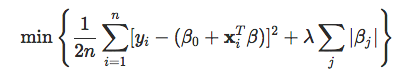(with standard notions, as in Wikipedia or Jocelyn Chi’s post – most of the code in this section is inspired by Jocelyn’s great post). Observe that the intercept is not subject to the penalty. The first order condition is then: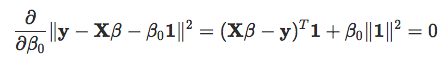Assume now that KKT conditions are satisfied since we cannot differentiate (to find points where the gradient is 0), we can check if contains the subdifferential at the minimum.Namely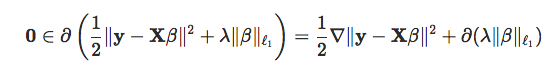For the term on the left, we recognize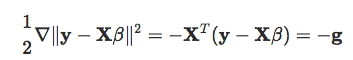so that the previous equation can be written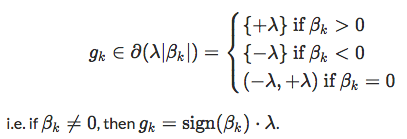Then we write the KKT conditions for this formulation and simplify them to produce a set of rules for checking our solution. We can split βj into a sum of its positive and negative parts by replacing βj with βj+−βj where βj+,βj−≥0. Then the Lasso problem becomes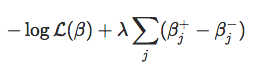with constraints βj+−βj. Let αj+,αj denote the Lagrange multipliers for -βj+,βj, respectively.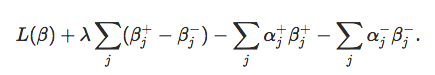To satisfy the stationarity condition, we take the gradient of the Lagrangian with respect to βj+ and set it to zero to obtain: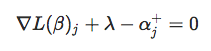We do the same with respect to βj to obtain: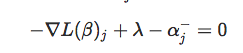As discussed in Jocelyn Chi’s post, primal feasibility requires that the primal constraints be satisfied so this gives us βj+≥0 and βj−≥0. Then dual feasibility requires non-negativity of the Lagrange multipliers so we get αj+≥0 and αj−≥0. And, finally, complementary slackness requires that αj+βj+=0 and αjβj−=0. We can simplify these conditions to obtain a simple set of rules for checking whether or not our solution is a minimum. The following is inspired by Jocelyn Chi’s post. From L(β)j+λαj+=0, we have L(β)j+λ=αj+≥0. This gives us L(β)j≥−λ. From L(β)j+λαj−=0, we have −∇L(β)j+λ=αj−≥0. This gives us −∇L(β)j≥−λ, which gives us L(β)jλ. Hence, ∣∇L(β)j∣≤λj When βj+>0,λ>0, complementary slackness requires αj+=0. So L(β)j+λ=αj+=0. Hence,L(β)j=−λ<0 since λ>0. At the same time, −∇L(β)j+λ=αj−≥0 so 2λ=αj−>0 since λ>0. Then complementary slackness requires βj−=0. Hence, when βj+>0, we have βj−=0 and L(β)j=−λ Similarly, when βj−>0,λ>0, complementary slackness requires αj−=0. So −∇L(β)j+λ=αj−=0 and L(β)j=λ>0 since λ>0. Then from L(β)j+λ=αj+≥0 and the above, we get 2λ=αj+>0. Then complementary slackness requires βj+=0. Hence, when βj−>0, we have βj+=0 and L(β)j=λ. Since βj=βj+−βj, this means that when L(β)j=−λ. And when βj<0L(β)j=λ. Combining this with ∣∇L(β)j∣≤λj, we arrive at the same convergence requirements that we obtained before using subdifferential calculus. For conveniency, introduce the soft-thresholding function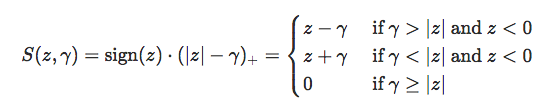Noticing that the optimization problem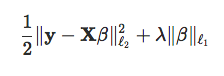can also be written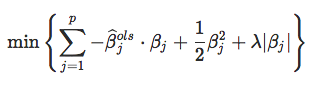observe that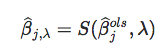which is a coordinate-wise update. Now, if we consider a (slightly) more general problem, with weights in the first part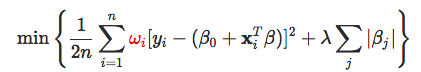the coordinate-wise update becomes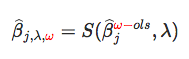An alternative is to set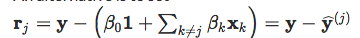so that the optimization problem can be written, equivalently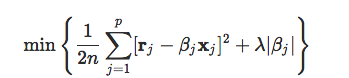hence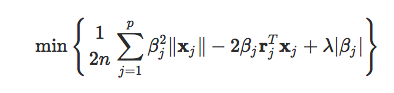and one gets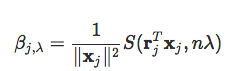or, if we develop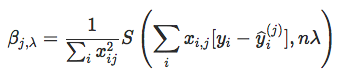Again, if there are weights ω=(ωi), the coordinate-wise update becomes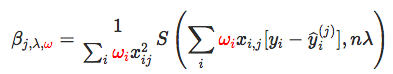The code to compute this componentwise descent is soft_thresholding = function(x,a){ result a)] a)] - a result[which(x &lt; -a)] = x[which(x &lt; -a)] + a return(result)} and the code lasso_coord_desc = function(X,y,beta,lambda,tol=1e-6,maxiter=1000){ beta = as.matrix(beta) X = as.matrix(X) omega = rep(1/length(y),length(y)) obj = numeric(length=(maxiter+1)) betalist = list(length(maxiter+1)) betalist[] = beta beta0list = numeric(length(maxiter+1)) beta0 = sum(y-X%*%beta)/(length(y)) beta0list = beta0 for (j in 1:maxiter){ for (k in 1:length(beta)){ r = y - X[,-k]%*%beta[-k] - beta0*rep(1,length(y)) beta[k] = (1/sum(omega*X[,k]^2))*soft_thresholding(t(omega*r)%*%X[,k],length(y)*lambda) } beta0 = sum(y-X%*%beta)/(length(y)) beta0list[j+1] = beta0 betalist[[j+1]] = beta obj[j] = (1/2)*(1/length(y))*norm(omega*(y - X%*%beta - beta0*rep(1,length(y))),'F')^2 + lambda*sum(abs(beta)) if (norm(rbind(beta0list[j],betalist[[j]]) - rbind(beta0,beta),'F') &lt; tol) { break } } return(list(obj=obj[1:j],beta=beta,intercept=beta0)) } Let’s keep that one warm, and let’s get back to our initial problem. ## The Lasso Logistic Regression The trick here is that the logistic problem can be formulated as a quadratic programming problem. Recall that the log-likelihood is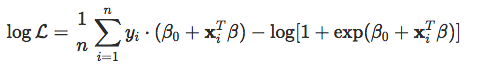which is a concave function of the parameters. Hence, one can use a quadratic approximation of the log-likelihood – using Taylor expansion: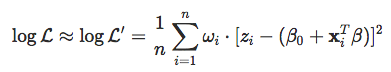where zi is the working response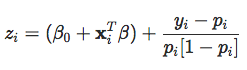pi is the prediction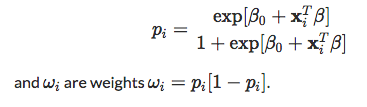Thus, we obtain a penalized least-square problem. And we can use what was done previously lasso_coord_desc = function(X,y,beta,lambda,tol=1e-6,maxiter=1000){ beta = as.matrix(beta) X = as.matrix(X) obj = numeric(length=(maxiter+1)) betalist = list(length(maxiter+1)) betalist[] = beta beta0 = sum(y-X%*%beta)/(length(y)) p = exp(beta0*rep(1,length(y)) + X%*%beta)/(1+exp(beta0*rep(1,length(y)) + X%*%beta)) z = beta0*rep(1,length(y)) + X%*%beta + (y-p)/(p*(1-p)) omega = p*(1-p)/(sum((p*(1-p)))) beta0list = numeric(length(maxiter+1)) beta0 = sum(y-X%*%beta)/(length(y)) beta0list = beta0 for (j in 1:maxiter){ for (k in 1:length(beta)){ r = z - X[,-k]%*%beta[-k] - beta0*rep(1,length(y)) beta[k] = (1/sum(omega*X[,k]^2))*soft_thresholding(t(omega*r)%*%X[,k],length(y)*lambda) } beta0 = sum(y-X%*%beta)/(length(y)) beta0list[j+1] = beta0 betalist[[j+1]] = beta obj[j] = (1/2)*(1/length(y))*norm(omega*(z - X%*%beta - beta0*rep(1,length(y))),'F')^2 + lambda*sum(abs(beta)) p = exp(beta0*rep(1,length(y)) + X%*%beta)/(1+exp(beta0*rep(1,length(y)) + X%*%beta)) z = beta0*rep(1,length(y)) + X%*%beta + (y-p)/(p*(1-p)) omega = p*(1-p)/(sum((p*(1-p)))) if (norm(rbind(beta0list[j],betalist[[j]]) - rbind(beta0,beta),'F') &lt; tol) { break } } return(list(obj=obj[1:j],beta=beta,intercept=beta0)) }It looks like what can get when calling glmnet… and here, we do have null components for some λ large enough ! Really null… and that’s cool actually. ## Application on Our Second Dataset Consider now the second dataset with two covariates. The code to get Lasso estimates is: df0 = df df0$y = as.numeric(df$y)-1 plot_lambda = function(lambda){ m = apply(df0,2,mean) s = apply(df0,2,sd) for(j in 1:2) df0[,j] &lt;- (df0[,j]-m[j])/s[j] reg = glmnet(cbind(df0$x1,df0$x2), df0$y==1, alpha=1,lambda=lambda)
u = seq(0,1,length=101)
p = function(x,y){
xt = (x-m)/s
yt = (y-m)/s
predict(reg,newx=cbind(x1=xt,x2=yt),type="response")}
v = outer(u,u,p)
image(u,u,v,col=clr10,breaks=(0:10)/10)
points(df$x1,df$x2,pch=19,cex=1.5,col="white")
points(df$x1,df$x2,pch=c(1,19)[1+z],cex=1.5)
contour(u,u,v,levels = .5,add=TRUE)}

Consider some small values, for [\lambda], so that we only have some sort of shrinkage of parameters:

reg = glmnet(cbind(df0$x1,df0$x2), df0$y==1, alpha=1) par(mfrow=c(1,2)) plot(reg,xvar="lambda",col=c("blue","red"),lwd=2) abline(v=exp(-2.8)) plot_lambda(exp(-2.8))But with a larger λ, there is variable selection: here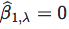reg = glmnet(cbind(df0$x1,df0$x2), df0$y==1, alpha=1)
par(mfrow=c(1,2))
plot(reg,xvar="lambda",col=c("blue","red"),lwd=2)
abline(v=exp(-2.1))
plot_lambda(exp(-2.1))(to be continued…)

Topics:
data classification ,big data ,lasso regression ,regression analysis ,r

Comment (0)

Save
{{ articles.views | formatCount}} Views

Published at DZone with permission of

Opinions expressed by DZone contributors are their own.